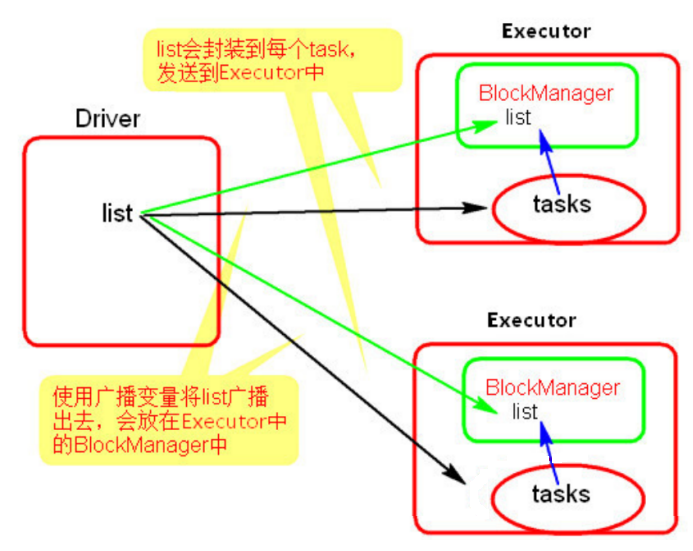# Spark Core 累加器、广播变量

10 篇文章 0 订阅

## Accumulator累加器（重要）

1. Spark提供了一个默认的累加器,只能用于求和没啥用

2. 如何使用:

2.1.通过SparkContext对象.accumulator(0) var sum = sc.accumulator(0)

​ 通过accumulator声明一个累加器,0为初始化的值

2.2.通过转换或者行动操作,通过sum +=n 来使用

2.3.如何取值? 在Driver程序中,通过 sum .value来获取值

3.累加器是懒执行,需要行动触发

 val numbers = sc .parallelize(List(1,2,3,4,5,6),2)
println(numbers.partitions.length)
//为什么sum值通过计算过后还是0
//因为foreach是没有返回值,整个计算过程都是在executor端完后
//foreach是在driver端运行所以打印的就是 0,foreach没有办法获取数据
//var sum = 0
//    numbers.foreach(num =>{
//      sum += num
//    })
//    println(sum)
//建议点击看原码 可以发现当前方法已经过时了,@deprecated("use AccumulatorV2", "2.0.0")
//所以以后使用时候需要使用自定义累加器
var sum = sc.accumulator(0)
numbers.foreach(num =>{
sum += num
})
println(sum.value)
}


### 自定义累加器

#### 案例1:已经定义好的数值类型可以直接使用

//这些类都是AccumulatorV2的子类可以直接使用
val conf = new SparkConf().setAppName("SparkWordCount").setMaster("local[*]")
//2.创建SparkContext 提交SparkApp的入口
val sc = new SparkContext(conf)
val num1 = sc.parallelize(List(1, 2, 3, 4, 5, 6), 2)
val num2 = sc.parallelize(List(1.1, 2.2, 3.3, 4.4, 5.5, 6.6), 2)
def longAccumulator(name: String): LongAccumulator = {
val acc = new LongAccumulator
sc.register(acc, name)
acc
}
val acc1 =  longAccumulator("kk")
println(acc1.value)

def doubleAccumulator(name: String): DoubleAccumulator = {
val acc = new DoubleAccumulator
sc.register(acc, name)
acc
}
val acc2 =  doubleAccumulator("kk")
println(acc2.value)



#### 案例2:

import org.apache.spark.{SparkConf, SparkContext}
import org.apache.spark.util.AccumulatorV2
//在继承的时候需要执行泛型 即 可以计算IN类型的输入值，产生Out类型的输出值
//继承后必须实现提供的方法
class MyAccumulator extends  AccumulatorV2[Int,Int]{
//创建一个输出值的变量
private var sum:Int = _

//必须重写如下方法:
//检测方法是否为空
override def isZero: Boolean = sum == 0
//拷贝一个新的累加器
override def copy(): AccumulatorV2[Int, Int] = {
//需要创建当前自定累加器对象
val myaccumulator = new MyAccumulator()
//需要将当前数据拷贝到新的累加器数据里面
//也就是说将原有累加器中的数据拷贝到新的累加器数据中
//ps:个人理解应该是为了数据的更新迭代
myaccumulator.sum = this.sum
myaccumulator
}
//重置一个累加器 将累加器中的数据清零
override def reset(): Unit = sum = 0
//每一个分区中用于添加数据的方法(分区中的数据计算)
override def add(v: Int): Unit = {
//v 即 分区中的数据
//当累加器中有数据的时候需要计算累加器中的数据
sum += v
}
//合并每一个分区的输出(将分区中的数进行汇总)
override def merge(other: AccumulatorV2[Int, Int]): Unit = {
//将每个分区中的数据进行汇总
sum += other.value

}
//输出值(最终累加的值)
override def value: Int = sum
}

object  MyAccumulator{
def main(args: Array[String]): Unit = {
val conf = new SparkConf().setAppName("MyAccumulator").setMaster("local[*]")
//2.创建SparkContext 提交SparkApp的入口
val sc = new SparkContext(conf)
val numbers = sc .parallelize(List(1,2,3,4,5,6),2)
val accumulator = new MyAccumulator()
//需要注册
sc.register(accumulator,"acc")
//切记不要使用Transformation算子 会出现无法更新数据的情况
//应该使用Action算子
//若使用了Map会得不到结果
println(accumulator.value)
}
}


#### 案例3:使用累加器做单词统计

import org.apache.spark.{SparkConf, SparkContext}
import org.apache.spark.util.AccumulatorV2

import scala.collection.mutable

class MyAccumulator2 extends AccumulatorV2[String, mutable.HashMap[String, Int]] {

private val _hashAcc = new mutable.HashMap[String, Int]()

// 检测是否为空
override def isZero: Boolean = {
_hashAcc.isEmpty
}

// 拷贝一个新的累加器
override def copy(): AccumulatorV2[String, mutable.HashMap[String, Int]] = {
val newAcc = new MyAccumulator2()
_hashAcc.synchronized {
newAcc._hashAcc ++= (_hashAcc)
}
newAcc
}

// 重置一个累加器
override def reset(): Unit = {
_hashAcc.clear()
}

// 每一个分区中用于添加数据的方法 小SUM
override def add(v: String): Unit = {
_hashAcc.get(v) match {
case None => _hashAcc += ((v, 1))
case Some(a) => _hashAcc += ((v, a + 1))
}

}

// 合并每一个分区的输出 总sum
override def merge(other: AccumulatorV2[String, mutable.HashMap[String, Int]]): Unit = {
other match {
case o: AccumulatorV2[String, mutable.HashMap[String, Int]] => {
for ((k, v) <- o.value) {
_hashAcc.get(k) match {
case None => _hashAcc += ((k, v))
case Some(a) => _hashAcc += ((k, a + v))
}
}
}
}
}
// 输出值
override def value: mutable.HashMap[String, Int] = {
_hashAcc
}
}
object MyAccumulator2 {
def main(args: Array[String]): Unit = {
val sparkConf = new SparkConf().setAppName("MyAccumulator2").setMaster("local[*]")
val sc = new SparkContext(sparkConf)
val hashAcc = new MyAccumulator2()
sc.register(hashAcc, "abc")
val rdd = sc.makeRDD(Array("a", "b", "c", "a", "b", "c", "d"),2)
for ((k, v) <- hashAcc.value) {
println("【" + k + ":" + v + "】")
}
sc.stop()

}

}


1.累加器的创建:

1.1.创建一个累加器的实例

1.2.通过sc.register()注册一个累加器

1.4.通过累加器实例名.value来获取累加器的值

2.最好不要在转换操作中访问累加器(因为血统的关系和转换操作可能执行多次),最好在行动操作中访问

1.能够精确的统计数据的各种数据例如:

### 问题说明:

/**

*/
val sc = new SparkContext(conf)
//list是在driver端创建也相当于是本地变量
val list = List("hello java")
//算子部分是在Excecutor端执行
val lines = sc.textFile("dir/file")
val filterStr = lines.filter(list.contains(_))
filterStr.foreach(println)executor的BlockManager除了从driver上拉取，也可能从其他节点的BlockManager上拉取变量副本。

BlockManager

[外链图片转存失败(img-6AfAWh0B-1565850929672)(./image/图片5.png)]

 val conf = new SparkConf().setAppName("BroadcastTest").setMaster("local")
val sc = new SparkContext(conf)
//list是在driver端创建也相当于是本地变量
val list = List("hello java")
//封装广播变量
//算子部分是在Excecutor端执行
val lines = sc.textFile("dir/file")
//使用广播变量进行数据处理 value可以获取广播变量的值
filterStr.foreach(println)


​ (2) 通过 value 属性访问该对象的值(在 Java 中为 value() 方法)。

​ (3) 变量只会被发到各个节点一次，应作为只读值处理(修改这个值不会影响到别的节点)。

10000M，500M，20倍。20倍~以上的网络传输性能消耗的降低；20倍的内存消耗的减少。

06-181672
03-05285301-2838
12-215449
09-07835
02-2316
05-23238
03-0352
06-2011
06-28515
03-05376
12-20145
10-121336
12-17162
02-20129
03-02449
02-202527
05-29106
11-2585
03-3146点击重新获取扫码支付余额充值